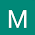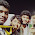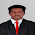Zero Power Factor (ZPF) Method

This method is also called potier method. In the operation of any alternator, the armature resistance drop and armature leakage reactance drop IXare actually e.m.f. quantities while the armature reaction is basically m.m.f. quantity. In the synchronous impedance all the quantities are treated as e.m.f. quantities as against this in M.M.F. method all are treated as m.m.f. quantities. Hence in both the methods, we are away from reality.
Note : This method is based on the separation of armature leakage reactance and armature reaction effects. The armature leakage reactance XL is called Potier reactance in this method, hence method is also called potier reactance method.
To determine armature leakage reactance and armature reaction m.m.f. separately, two tests are performed on the given alternator. The two tests are,
1. Open circuit test
2. Zero power factor test
1.1 Open Circuit Test
The experimental setup to perform this test is shown in the Fig. 1.Fig. 1
The steps to perform open circuit test are,
1. The switch S is kept open.
2. The alternator is driven by its prime mover at its synchronous speed and same is maintained constant throughout the test.
3. The excitation is varied with the help of potential divider, from zero upto rated value in definite number of steps. The open circuit e.m.f. is measured with the help of voltmeter. The readings are tabulated.
4. A graph of If and (Voc) i.e. field current and open circuit voltage per phase is plotted to some scale. This is open circuit characteristics.
1.2 Zero Power Factor Test
To conduct zero power factor test, the switch S is kept closed. Due to this, a purely inductive load gets connected to an alternator through an ammeter. A purely inductive load has power factor of cos i.e. zero lagging hence the test is called zero power factor test.
The machine speed is maintained constant at its synchronous value. The load current delivered by an alternator to purely inductive load is maintained constant at its rated full load value by varying excitation and by adjusting variable inductance of the inductive load. Note that, due to purely inductive load, an alternator will always operate at zero p.f. lagging.
Note : In this test, there is no need to obtain number of points to obtain the curve. Only two points are enough to construct a curve called zero power factor saturation curve.
This is the graph of terminal voltage against excitation when delivering full load zero power factor current.
One point for this curve is zero terminal voltage (short circuit condition) and the field current required to deliver full load short circuit armature current. While other point is the field current required to obtain rated terminal voltage while delivering rated full load armature current. With the help of these two points the zero p.f. saturation curve can be obtained as,
1. Plot open circuit characteristics on graph as shown in the Fig. 2.Fig. 2
2. Plot the excitation corresponding to zero terminal voltage i.e. short circuit full load zero p.f. armature current. This point is shown as A in the Fig. 1 which is on the x-axis. Another point is the rated voltage when alternator is delivering full load current at zero p.f. lagging. This point is P as shown in the Fig. 1.
3. Draw the tangent to O.C.C. through origin which is line OB as shown dotted in the Fig. 1. This is called air line.
4. Draw the horizontal line PQ parallel and equal to OA.
5. From point Q draw the line parallel to the air line which intersects O.C.C. at point R. Join RQ and join PR. The triangle PQR is called potier triangle.
6. From point R, drop a perpendicular on PQ to meet at point S.
7. The zero p.f. full load saturation curve is now be constructed by moving a triangle PQR so that R remains always on O.C.C. and line PQ always remains horizontal. The doted triangle is shown in the Fig. 1. It must be noted that the potier triangle once obtained is constant for a given armature current and hence can be transferred as it is.
8. Through point A, draw line parallel to PR meeting O.C.C. at point B. From B, draw perpendicular on OA to meet it at point C. Triangles OAB and PQR are similar triangles.
9. The perpendicular RS gives the voltage drop due to the armature leakage reactance i.e. IXL.
10. The length PS gives field current necessary to overcome demagnetising effect of armature reaction at full load.
11. The length SQ represents field current required to induce an e.m.f. for balancing leakage reactance drop RS.
These values can be obtained from any Potier triangle such as OAB, PQR and so on.
This is nothing but the potier reactance.
1.3 Use of Potier Reactance to Determine Regulation
To determine regulation using Potier reactance, draw the phasor diagram using following procedure :
Draw the rated terminal voltage Vph as a reference phasor. Depending upon at which power factor (cosΦ) the regulation is to be predicted, draw the Current phasor Iph lagging or leading Vph by angle Φ.
Draw Iph Raph voltage drop to Vph which is in phase with Iph. While the voltage drop Iph XLph is to be drawn perpendicular to Iph Raph vector but leading Iph Raph at the extremely of Vph.
The Raph is to be measured separately by passing a d.c. current and measuring voltage across armature winding. While XLph is Potier reactance obtained by Potier method.
Phasor sum of Vph rated, Iph Raph and Iph XLph gives the e.m.f. which is say E1ph.

Obtain the excitation corresponding to Ē1ph from O.C.C. drawn. Let this excitation be Ff1. This is excitation required to induce e.m.f. which does not consider the effect of armature reaction.
The field current required to balance armature reaction can be obtained from Potier triangle, which is say FAR.
...     FAR l (PS) =  l (AC) =  .....
The total excitation required is the vector sum of the Ff1 and FAR. This can be obtained exactly similar to the procedure used in M.M.F. method.
Draw vector Ff1 to some scale, leading E1ph by 90o. Add FAR to Ff1 by drawing vector FAR in phase opposition to Iph. The total excitation to be supplied by field is given by FR
The complete phasor diagram is shown in the Fig. 3.Fig. 3
Once the total excitation is known which is FR, the corresponding induced e.m.f. Eph can be obtained from O.C.C. This Eph lags FR by 90o. The length CD represents voltage drop due to the armature reaction. Drawing perpendicular from A and B on current phasor meeting at points G and H respectively, we get triangle OHC as right angle triangle. Hence E1ph can be determined analytically also.
Once Eph is known, the regulation of an alternator can be predicted as,

This method takes into consideration the armature resistance an leakage reactance voltage drops as e.m.f. quantities and the effect of armature reaction as m.m.f. quantity. This is reality hence the results obtained by this method are nearer to the reality than those obtained by synchronous impedance method and ampere-turns method.
The only drawback of this method is that the separate curve for every load condition is necessary to plot if potier triangles for various load conditions are required.

Examples on Zero Power Factor (ZPF) Method

1.Hi there! glad to drop by your page and found these very interesting and informative stuff. Thanks for sharing, keep it up!

- p-f curve

2.3.thanks...................good explanation

4.Thanks for sharing this valuable info.. with us :)

5.This comment has been removed by the author.

6.Where is therotical explation

7.This helped me. Thanks.

8.you mentioned that pqr is potire triangel but upon my knowledge it would we prs is potier triangel because that triangel does nt consitst of leakag reactance graphically otherwise ur explanation is awesome

9.IN ZPF method If=Ifg-Ifa but in EMF and MMF method it is If=Ifg+Ifa. Why it should be differance in ZPF method?

1.Because in potier method we consider IaRa & IaXl as part of E.M.F & IaXs as part of M.M.F.

10.11.How to perform zpfc test when specified er factor is 0.9

12.Is it possible to find regulation by connecting pure capacitive load